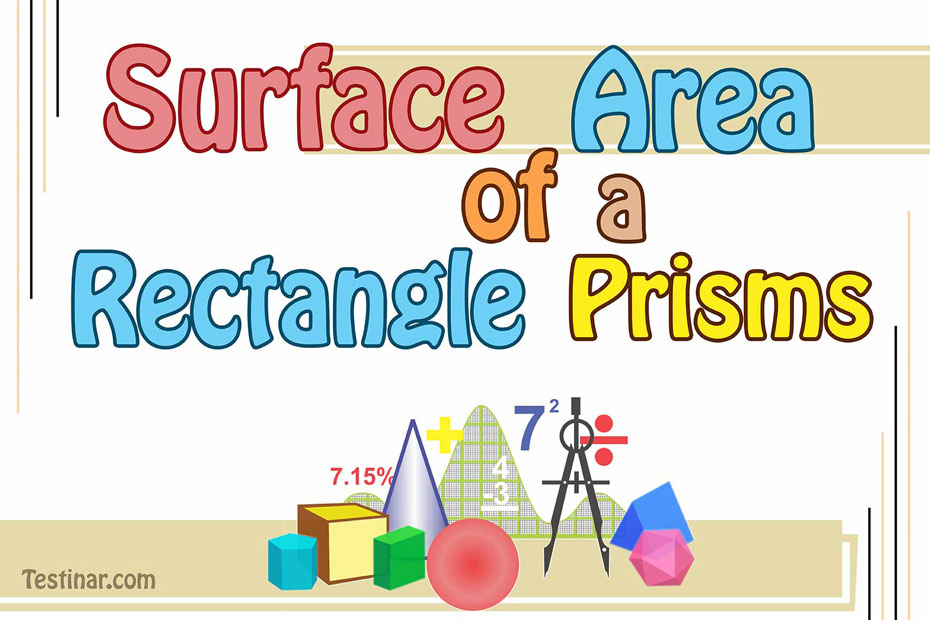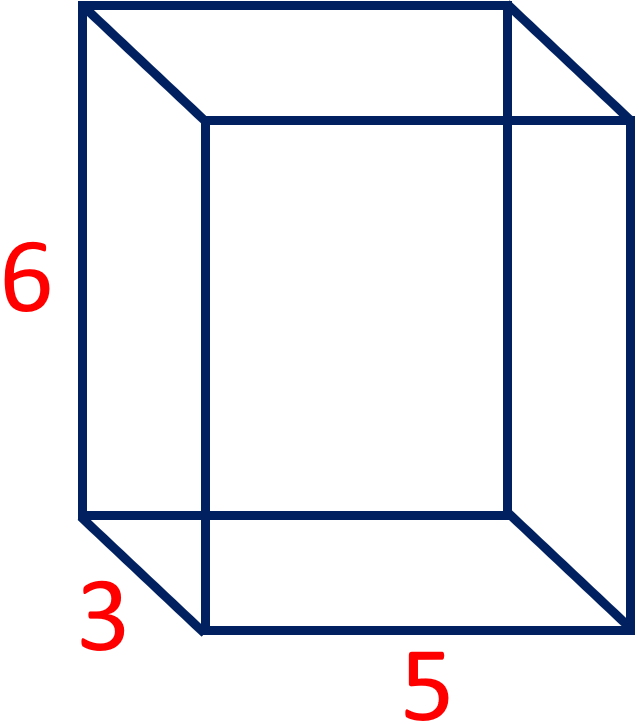## How to Find the Volume of a Rectangular Prism

### The volume of Rectangular Prisms

A prism is a polyhedron with flat sides and bases of the same length. It is a solid object with flat sides, the same ends, and a cross-section that is the same length as the object. In Geometry, we will learn about the different prisms, such as a triangular prism, a pentagonal prism, and a hexagonal prism. Because it is a three-dimensional figure, it has both a surface area and a volume.

### How much space does a prism take up?

The volume of a prism is the amount of space the three-dimensional object takes up as a whole. In math, it is equal to the product of the length and the area of the base. Therefore,

The volume of a prism $$=$$ Base Area $$\times$$ Length

A three-dimensional object's volume is expressed in cubic units, a measuring unit.

### The volume of a rectangle-shaped prism

A rectangular prism has four straight sides and two square bases next to each other. We know that a rectangular prism is cut into a rectangle. The square-shaped prism is also called a "cuboid."

So, here is the formula for figuring out the volume of a rectangular prism:

A rectangular prism has a volume of $$l \times b \times h$$ cubed units. Where:

• $$l \ =$$ Width of a rectangle's base
• $$b \ =$$ length of the base of a rectangular prism
• $$h \ =$$ the height of a rectangle

### Example:

Find the volume of the rectangle-shaped prism shown below.• Step 1: Figure out the rectangular prism's length $$(l)$$, width $$(w)$$, and height $$(h)$$.
Most of the time, the vertical side of a rectangular prism is thought to be its height, so $$h \ = \ 6$$ cm. Length is usually believed to be the length of the remaining measurements, so we have $$l \ = \ 5$$ cm. and $$w \ = \ 3$$ cm.
• Step 2: Use the formula $$V \ = \ l \times w \times h$$ to figure out the volume. Don't forget to put the units, units$$^3$$.
To get the volume, we need to multiply the length, width, and height.
$$V \ = \ 5 \times 3 \times 6 \ = \ 90$$

The volume is $$90$$ cm$$^3$$.

### Exercises for Volume of Rectangle Prisms

1)2)3)4)5)6)7)8)9)10)1) $$\color{red}{48}$$ m$$^3$$2) $$\color{red}{6840}$$ m$$^3$$3) $$\color{red}{1859}$$ mm$$^3$$4) $$\color{red}{120}$$ in$$^3$$5) $$\color{red}{7980}$$ in$$^3$$6) $$\color{red}{2028}$$ cm$$^3$$7) $$\color{red}{3315}$$ m$$^3$$8) $$\color{red}{10080}$$ m$$^3$$9) $$\color{red}{280}$$ m$$^3$$10) $$\color{red}{480}$$ mm$$^3$$## Volume of Rectangle Prisms Practice Quiz

### Praxis Core Math Full Study Guide

$25.99$13.99

### TSI Math for Beginners 2022

$24.99$14.99

### CHSPE Math Full Study Guide

$25.99$13.99

### The Most Comprehensive CHSPE Math Preparation Bundle

$76.99$36.99